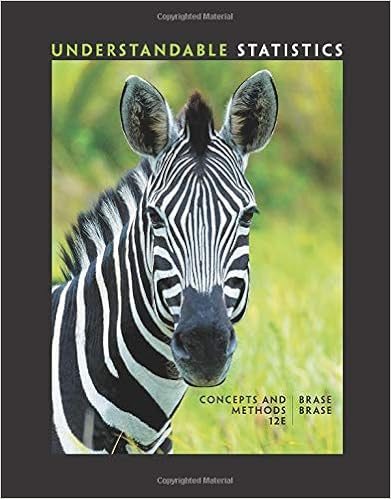# The study of statistics encompasses all of the following...

• Notes
• 12

This preview shows page 1 - 3 out of 12 pages.

##### We have textbook solutions for you!
The document you are viewing contains questions related to this textbook.The document you are viewing contains questions related to this textbook.
Chapter 6 / Exercise 3
Understandable Statistics: Concepts and Methods
BraseExpert Verified
Practice questions for Exam 1 This practice exam is not intended to duplicate the actual exam. It is intended to provide practice as part of your exam preparation. 1. The study of statistics encompasses all of the following except A. properly collecting of data. B. organizing and summarizing data. C. correctly obtaining anecdotal data. D. drawing inferences from sample data.
2. Which one of the following will change the appearance of a histogram?
3. A company gives yearly performance ratings to each of its employees. These ratings are E for exemplary performance, A for acceptable performance and I for performance needing improvement. The 24 employees in the receiving department were given the following ratings: A A A E I E E A E A I A A E E A What type of graphical display should you use for this data? E A A I A I E E
4. For the data in the previous question, what type of numerical summary (summaries) is (are) appropriate?
5. A professor wishes to graphically display the exam scores for 18 students in his class. The scores ranged from 45% to 100%. You should recommend that he use A. a histogram B. a stem and leaf plot C. a bar graph D. a pie chart.
6. Which one of the following variables is categorical (also called qualitative)?
##### We have textbook solutions for you!
The document you are viewing contains questions related to this textbook.The document you are viewing contains questions related to this textbook.
Chapter 6 / Exercise 3
Understandable Statistics: Concepts and Methods
BraseExpert Verified
7. Which one of the following variables is quantitative (also called numerical)?
8. Which of the following is NOT a measure of center of the data?
9. When the data are arranged in ascending numerical order, the median is the value which A. cuts the data in half. B. "balances" the data. C. occurs the most frequently. D. gives an appropriate measure of the spread of the data.
10. The five number summary consists of the following five numbers: + 2s
•••